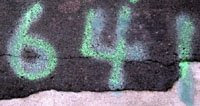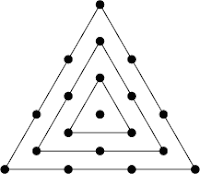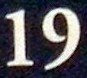## Friday, February 27, 2009

### 632

632 = 23 x 79.

632 is a happy number. Take the sum of the squares of the digits of a number. Repeat the operation. If the result is eventually 1, the original number is happy: 62 + 32 + 22 = 49; 42 + 92 = 97; 92 + 72 = 130; 12 + 32 + 02 = 10; 12 + 02 = 1.

632 is the number of different necklaces (that can't be turned over) possible with 13 beads, each bead being one of 2 colors.

632 is a member of the following sequence: Start with 1 and repeatedly reverse the digits and add 7 to the next term: 1, 8, 15, 58, 92, 36, . . . 526, 632, 243., . . .The prophet Muhammad, founder of Islam, died in the year 632.

Source: Number Gossip

## Thursday, February 26, 2009

### 219

219 = 3 x 73.

219 is the smallest number with two representations as a sum of four positive cubes: 219 = 13 + 13 + 13 + 63 = 33 + 43 + 43 + 43.

The number
219n + 2 is prime for the smallest three primes (2, 3, 5): 2192 + 2 = 47963, 2193 + 2 = 10503461, 2195 + 2 = 503756397101.

219 is 11011011 in base 2 (binary) and 333 in base 8.219 is the number of space groups in three dimensions, analogous to the 17 basic wallpaper patterns in two dimensions, without considering handedness. When including handed forms, they determine the possible shapes of mineral crystals.

Source:
The Penguin Dictionary of Curious and Interesting Numbers, rev. ed., by David Wells.

## Wednesday, February 25, 2009

### 220

220 = 22 x 5 x 11.

220 is the 10th tetrahedral number.

220 and 284 form the first and smallest pair of amicable numbers. Each is the sum of the aliqot parts of the other. The aliquot parts of 220 are 1, 2, 4, 5, 10, 11, 20, 22, 44, 55, and 110 and total 284. 284 = 22 x 71 and its aliquot parts are 1, 2, 4, 71, and 142, totalling 220.

220 is a number that cannot be written as a sum of three squares.In the Bible,
220 was the number of goats Jacob gave to his brother Esau ("two hundred she-goats and twenty he-goats") and the number of sheep ("two hundred ewes and twenty rams," Genesis 32:14).

Source:
VirtueScience.com

## Tuesday, February 24, 2009

### 239

239 is a prime number. It is a Sophie Germain prime because 2 x 239 + 1 = 479, which is also a prime.

239 and 241 form a twin prime pair.

239 = 2 x 43 + 4 x 33 + 3 x 13.

239 and 23 are the only numbers that cannot be represented in fewer than nine positive cubes (the maximum).

239 needs four squares (the maximum) to express it and 19 fourth powers (the maximum) to express it. However, 239 doesn't need the maximum number of fifth powers.

The largest prime factor of
n2 + 1 is at least 17, provided n is greater than 239.The movie
Pu-239, named for a radioactive isotope of the element plutonium, concerns a Russian nuclear plant worker.

Source:
Wolfram MathWorld

## Monday, February 23, 2009

### 251

251 is a prime number. It is also a Sophie Germain prime because 2 x 251 + 1 = 503 is also prime.

251 is the smallest number that is the sum of three different cubes in two ways: 251 = 13 + 53 + 53 = 23 + 33 + 63.

251 can be expressed using the first three primes: 251 = 23 + 33.

251 is a divisor of 205 - 1.Telephone area code
251 covers southwestern Alabama.

Source:
Prime Curios!

## Friday, February 20, 2009

### 641

641 is a prime number. It is a Sophie Germain prime because 2 x 641 + 1 = 1283, which is also prime.

Leonhard Euler found the first counterexample to Fermat's conjecture that 22^n + 1 is always prime, when he discovered in 1742 that 22^5 + 1 is divisible by 641. All factors of 22^n + 1 are of the form k x 2n + 1 + 1. In this case 641 = 10 x 26 + 1.

641 has a representation as a sum of two squares: 641 = 42 + 252.

641 and 643 form a twin prime pair.

641 is the hypotenuse of a primitive Pythagorean triple: 6412 = 2002 + 6092.The telephone area code 641 covers the central portion of Iowa.

Source: D. Wells. 1997. The Penguin Dictionary of Curious and Interesting Numbers, rev. ed.

## Thursday, February 19, 2009

### 1001

1001 = 7 x 11 x 13.

To test a number for divisibility by any of the three divisors 7, 11, and 13 simultaneously, start by marking off the number to be tested in groups of three digits from the units position. Add the first, third, fifth, . . . groups and take away the total of the second, fourth, . . . groups. The number will be divisible by 7, 11, or 13 if the result is divisble by 7, 11, or 13 respectively.

1001 is the 11th pentatope number.

1001 is the fourth palindromic pentagonal number. The sequence of these numbers runs: 1, 5, 22, 1001, 2882, 15,251, . . .In many cases, including the title
One Thousand and One Arabian Nights, 1001 is meant to indicate a "big number" and need not be taken literally.

Source:
The Penguin Dictionary of Curious and Interesting Numbers, rev. ed., by David Wells.

## Wednesday, February 18, 2009

### 75

75 = 3 x 52

75 is the smallest pandigital number in base 4: 75 is written as 1023 in base 4 and contains all the possible digits.

75 is 55 in base 14.

275 + 75 is a prime number.

Take the "digit sum" 7 + 5 = 12, then continue adding in pairs (as shown below). You reach 75.
7 + 5 = 12
5 + 12 = 17
12 + 17 = 29
17 + 29 = 46
29 + 46 = 75Messier object M75 is a globular cluster in the constellation Sagittarius.

Source: Number Gossip

## Tuesday, February 17, 2009

### 123

123 = 3 x 41.

123 is the 10th Lucas number.

123 x 2123 - 1 is a prime number.

1234
2 - 1232 - 456 is a prime number.

123 is a number of the form n2 + 2, where n = 11.The STS-
123 space shuttle mission delivered the Japanese Kibo Logistics Module and the Canadian Dextre robotics system to the International Space Station in 2007.

Source:
Prime Curios!

## Friday, February 13, 2009

### 2101

2101 = 11 x 191.

2101 is a number n such that n, n + 1, and n + 2 are each the product of two primes: 2101 = 11 x 191, 2102 = 2 x 1051, 2103 = 3 x 701.

2101 is a number that yields a prime whenever a 1 is inserted anywhere in them, including the beginning or the end: 12101, 21101, and 21011 are all primes.2101 Adonis was one of the first near-Earth asteroids to be discovered.

Source:
On-Line Encyclopedia of Integer Sequences

## Thursday, February 12, 2009

### 920

920 = 23 x 5 x 23.

920 is a truncated cube number.

920 is a number n such that n2 is the sum of two successive primes: 9202 = 846,400 = 423,191 + 423,209.

920 is the maximum number of regions into which 18 triangles divide the plane.Telephone area code 920 covers Milwaukee, Wisconsin.

## Wednesday, February 11, 2009

### 192

192 = 26 x 3.

192 + 384 = 576, a pandigital sum where 384 = 2 x 192 and 576 = 3 x 192. There are three other such sums, starting with 219, 273, and 327.

192 is the smallest number that together with its double and triple contain every digit from 1 to 9 exactly once: 192, 384, and 576.

192 has a unique representation as a sum of three squares: 192 = 82 + 82 + 82.

192 is the number of integer partitions of 27 into distinct parts.192 Nausikaa is a large main belt asteroid.

Source:
Number Gossip

## Tuesday, February 10, 2009

### 34

34 = 2 x 17.

34 is the ninth Fibonacci number.

34 is the smallest number with the property that it and its neighbors have the same number of divisors (four): 33 = 3 x 11; 34 = 2 x 17; 35 = 5 x 7.

The rows and columns of a 4 x 4 magic square always total
34.

34 has a representation as a sum of two squares: 34 = 32 + 52.

34 is the smallest number with four representations as a sum of two primes: 34 = 3 + 31 = 5 + 29 = 11 + 23 = 17 + 17.

34 is 22 in base 16.

34 is a heptagonal number.34 is the atomic number of the element selenium.

## Monday, February 9, 2009

### 726

726 = 2 x 3 x 112.

726 is the start of a record-breaking run (smallest set) of six consecutive integers (from 726 to 731) with a distinct number of prime factors (from 1 to 6).

726 is 1011010110 in base (binary), 10401 in base 5, 600 in base 11, and 141 in base 25.

726 is the sum of nonconsecutive factorial numbers: 726 = 3! + 6! = 6 + 720.Introduced in 1952, the IBM 726 was one of the first practical high-speed magnetic tape systems for electronic digital computers.

## Friday, February 6, 2009

### 92

92 = 22 x 23.

92 is a pentagonal number.

92 is the maximum number of regions into which 13 lines divide a plane.

92 is the maximum number of regions into which 10 circles divide a plane.

92 is a number that cannot be written as a sum of three squares.The snub dodecahedron is an Archimedean solid with 92 faces.Source: Wolfram MathWorld

## Thursday, February 5, 2009

### 501

501 = 3 x 167.

501 is the sum of the first 18 prime numbers.

501 is the number of partitions of five items into ordered lists.

501 is the sum of 11 positive fifth powers: 501 = 35 + 25 + 25 + 25 + 25 + 25 + 25 + 25 + 25 + 15 + 15.Telephone area code 501 covers Little Rock, Arkansas.

## Wednesday, February 4, 2009

### 3079

3079 is a prime number.

3079 is a number that cannot be written as a sum of three squares.

3079 is a prime of the form n2 - n - 1, for n = 56.

3079 is a prime p such that p - 1 and p + 1 are divisible by cubes: 3078 = 2 x 34 x 19 and 3080 = 23 x 5 x 7 x 11.NGC
3079 is a spiral galaxy, found in the direction of the constellation Ursa Major about 55 million light years from Earth.Chandra X-ray Observatory image of NGC 3079.

Source: Chandra X-ray Observatory

Image: NASA/CXC/U.North Carolina/G.Cecil

## Tuesday, February 3, 2009

### 187

187 = 11 x 17.

The
187th prime number is 1117, which can be written with the digits of 187. This is the smallest prime with this property.

187 = 59 + 61 + 67 (sum of three consecutive primes).

187 = 7 + 11 + 13 + 17 + 19 + 23 + 29 + 31 + 37 (sum of 9 consecutive primes).

187 = 23 + 33 + 33 + 53 (sum of cubes).187 is the number of odd days in a leap year.

Source:
Prime Curios!

## Monday, February 2, 2009

### 19

19 is a prime number.

19 has a unique representation as a sum of three squares: 19 = 12 + 32 + 32.

19 is the fourth centered triangular number.19 is the largest prime number that is a palindrome in Roman numerals (XIX).

17 and 19 form a twin prime pair.Go is played by two players who alternately place black and white pieces on the vacant intersections of a grid of 19 by 19 lines.

Source:
Number Gossip